# Colon

## Introduction

The colon can be divided into six segments both anatomically and endoscopically. Unlike in the stomach, the histology of the colon is relatively uniform. These segments can be divided into the cecum, where the ileocecal valve and appendiceal orifice serve as important landmarks; ascending colon; transverse colon; descending colon; sigmoid colon; and rectum. Appreciation of the endoscopic differences between regions is important, particularly when dealing with colonic neoplasms, where accurate localization is essential.

In contrast with the upper gastrointestinal tract, in the colon, diagnosis and therapy of neoplasms assume a prominent role. Although adenomatous polyps are the most frequent neoplastic lesions, a variety of other polyps may masquerade endoscopically; subtle mucosal differences may aid in distinguishing these impostors. Inflammatory disorders such as Crohn’s disease and ulcerative colitis represent another important group of diseases. Other inflammatory disorders, including ischemia and infections (bacterial and viral), assume greater importance in the colon than in the upper gastrointestinal tract. Many of these inflammatory processes appear endoscopically similar; however, differentiation can usually be accomplished based on the characteristics of the patient, location of disease (pancolonic versus segmental), and characteristics of the disease in the involved segment (e.g., circumferential versus patchy; ulcer versus no ulcer).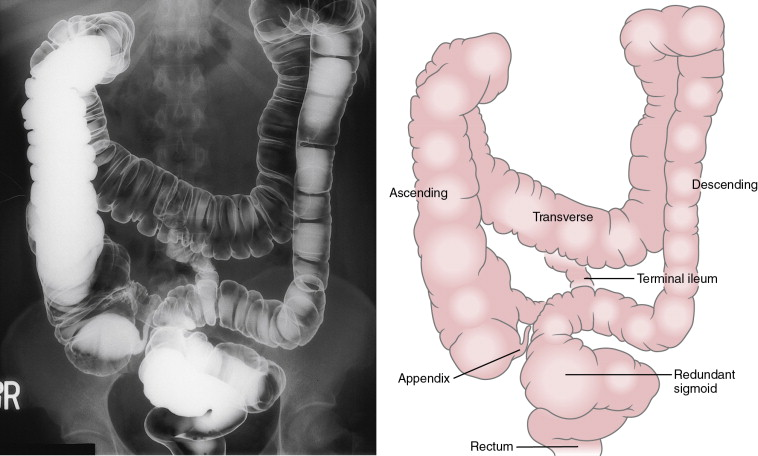Figure 5.1Figure 5.2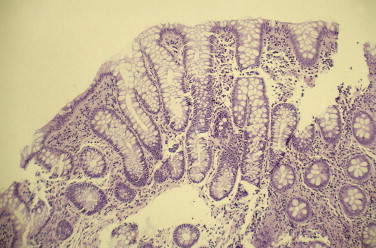Figure 5.3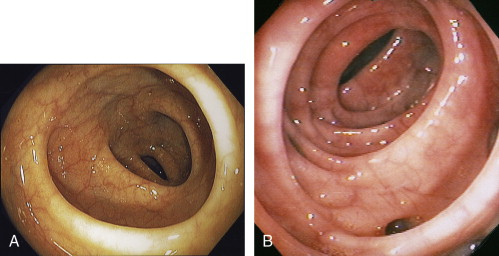Figure 5.4Figure 5.5Figure 5.6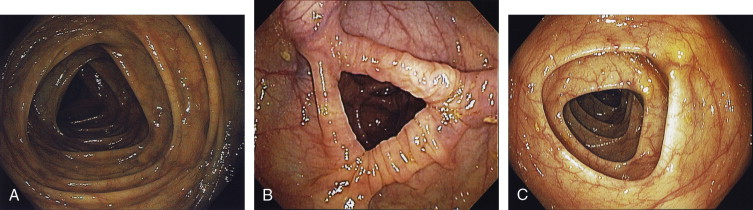Figure 5.7Figure 5.8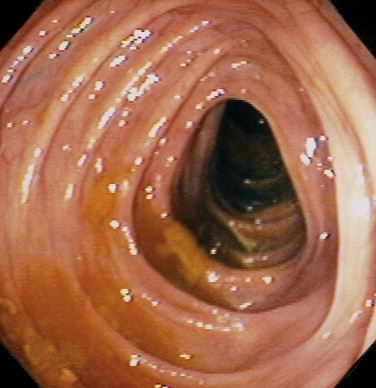Figure 5.9Figure 5.10Figure 5.11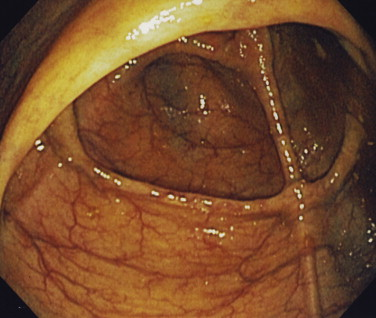Figure 5.12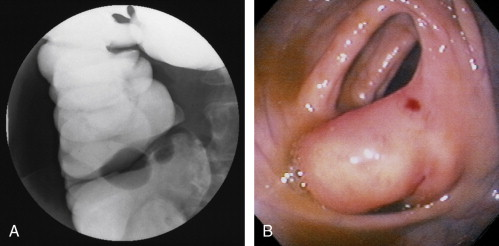Figure 5.13Figure 5.14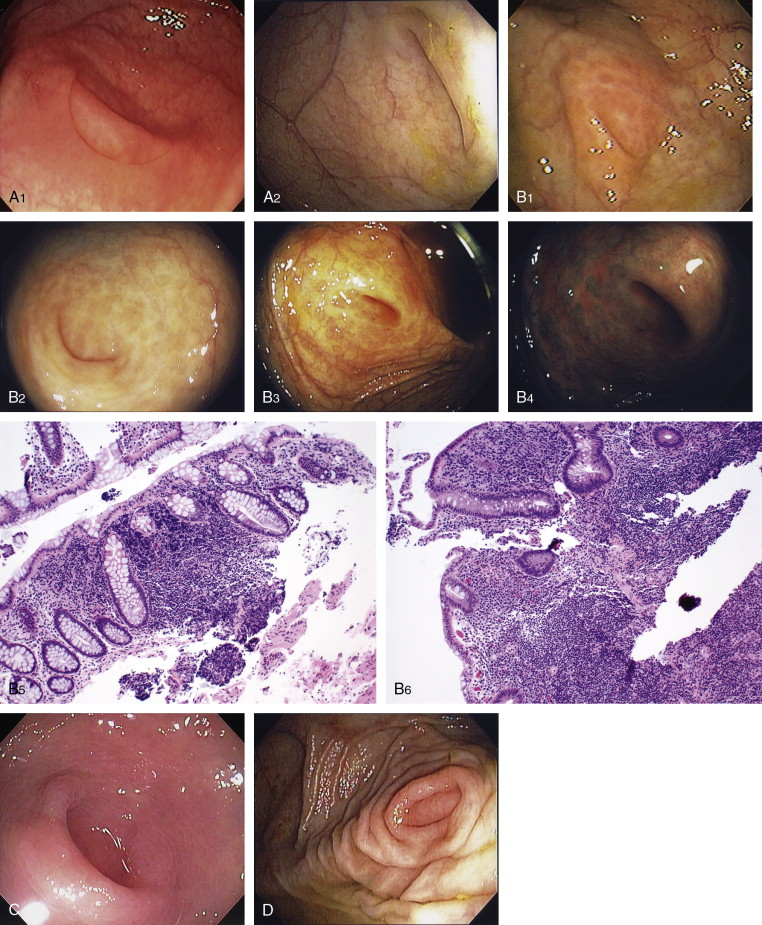Figure 5.15Figure 5.16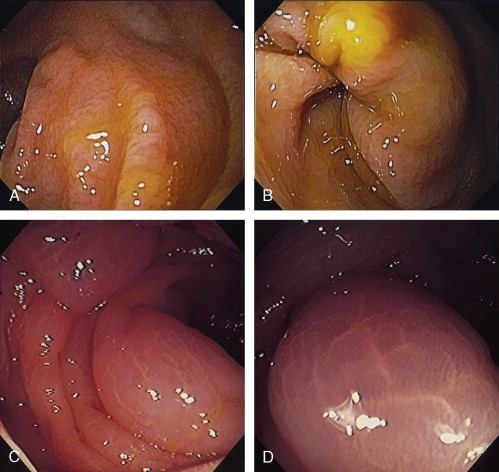Figure 5.17Figure 5.18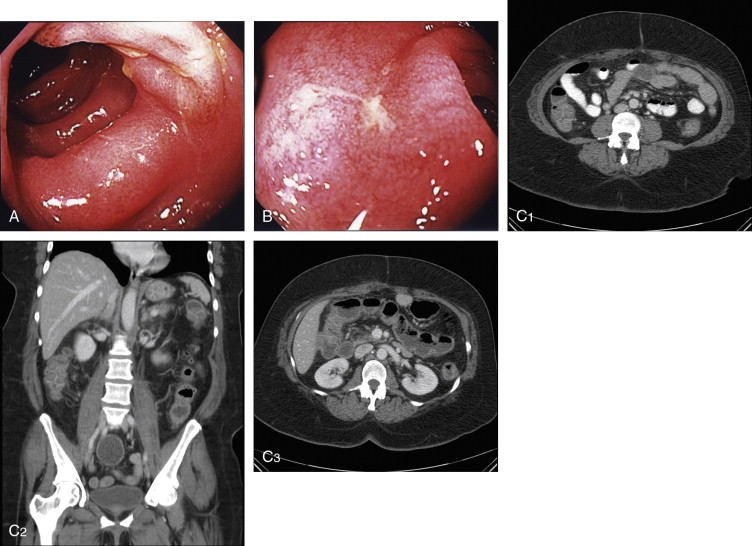Figure 5.19

Campylobacter Colitis ( Figure 5.20 )

• Inflammatory bowel disease, ulcerative colitis

• Ischemia

• Other infections (bacterial, viral)Figure 5.20

Differential Diagnosis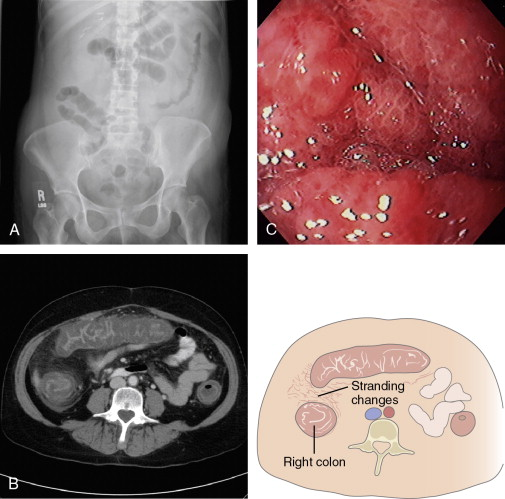Figure 5.21

Clostridium difficile Colitis ( Figure 5.22 )

• Ischemic colitis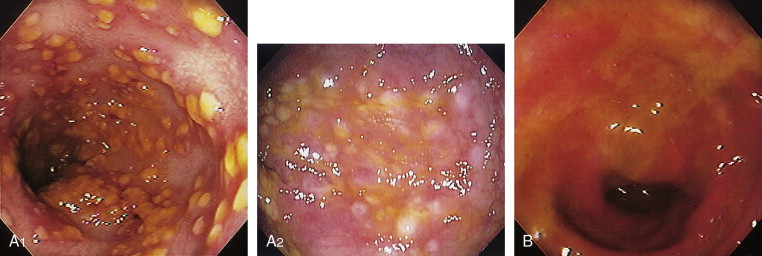Figure 5.22

Differential Diagnosis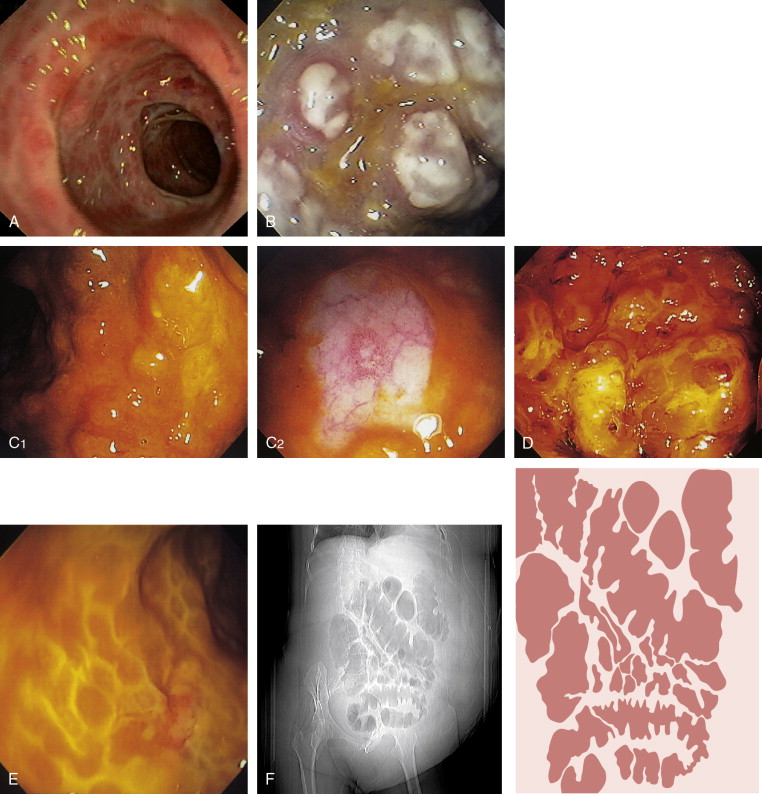Figure 5.23Figure 5.24Figure 5.25Figure 5.26

Cytomegalovirus Colitis ( Figure 5.27 )

• Inflammatory bowel disease

• Other infections

• IschemiaFigure 5.27

Differential DiagnosisFigure 5.28Figure 5.29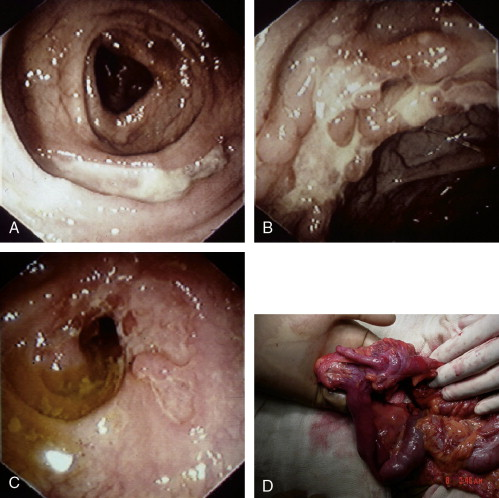Figure 5.30Figure 5.31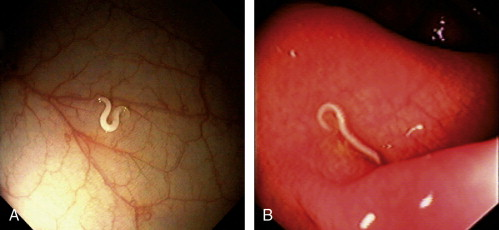Figure 5.32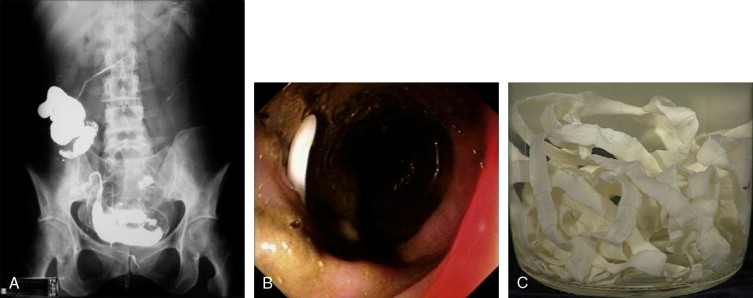Figure 5.33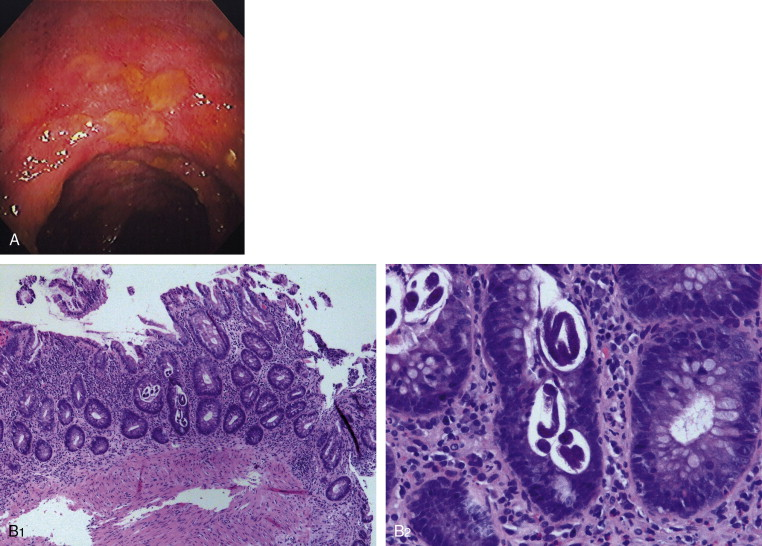Figure 5.34

Amebic Colitis ( Figure 5.35 )

• Inflammatory bowel disease

• Other infections (viral, bacterial)Figure 5.35

Differential Diagnosis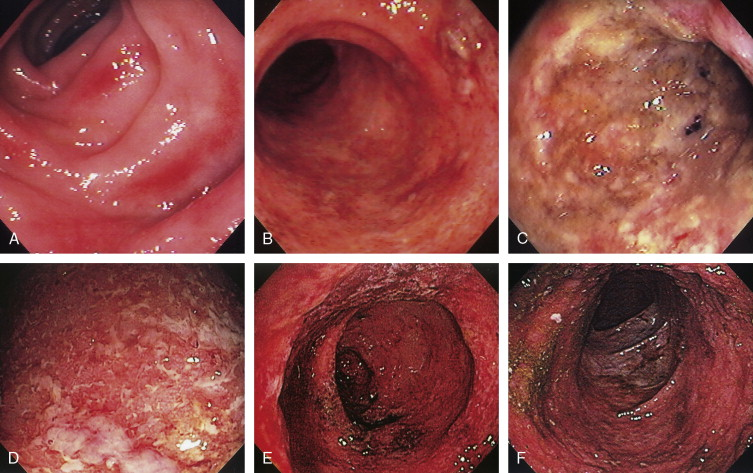Figure 5.36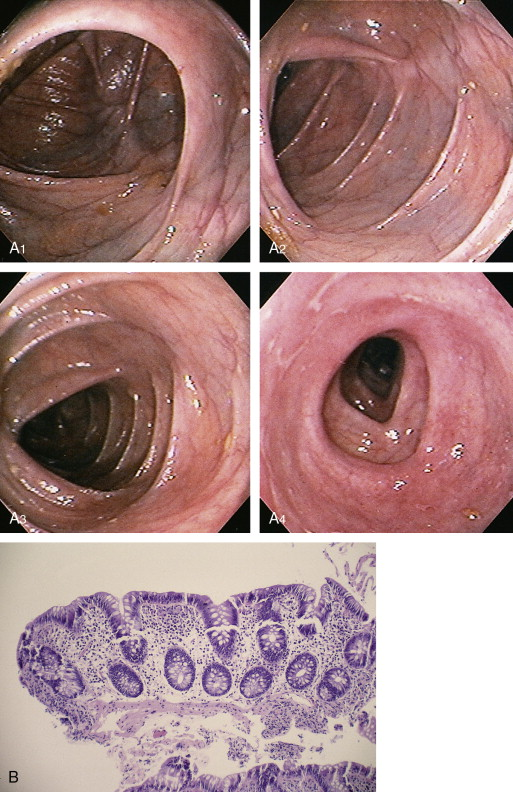Figure 5.37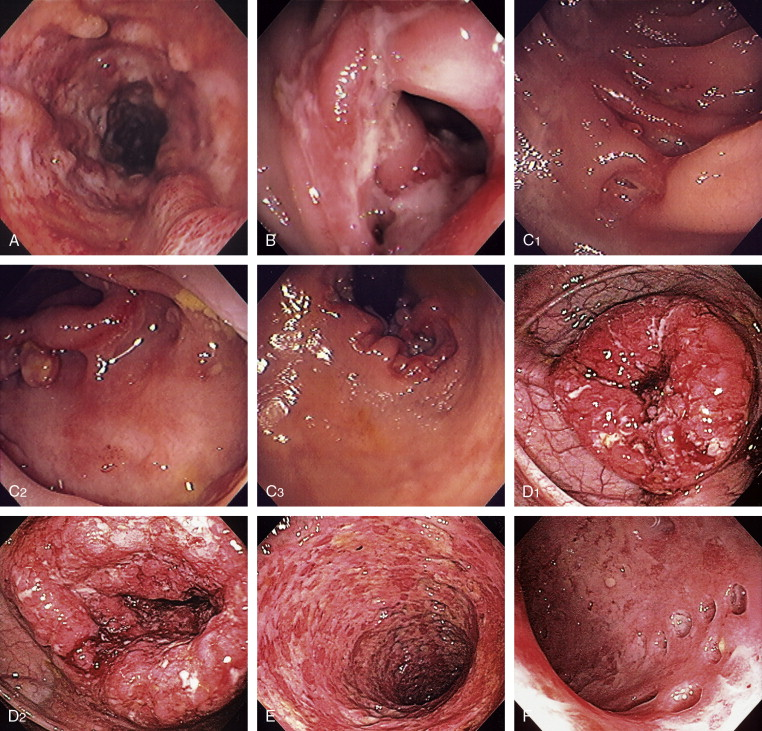Figure 5.38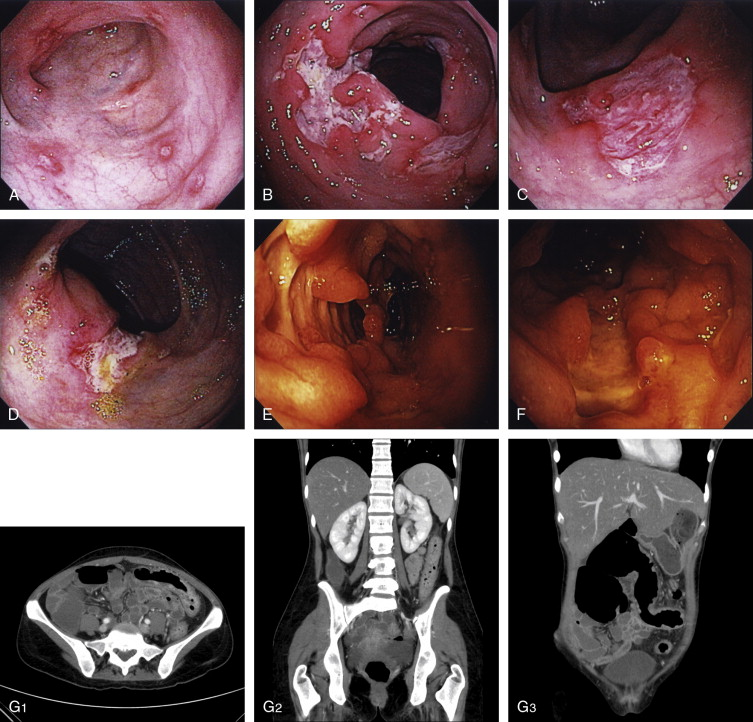Figure 5.39Figure 5.40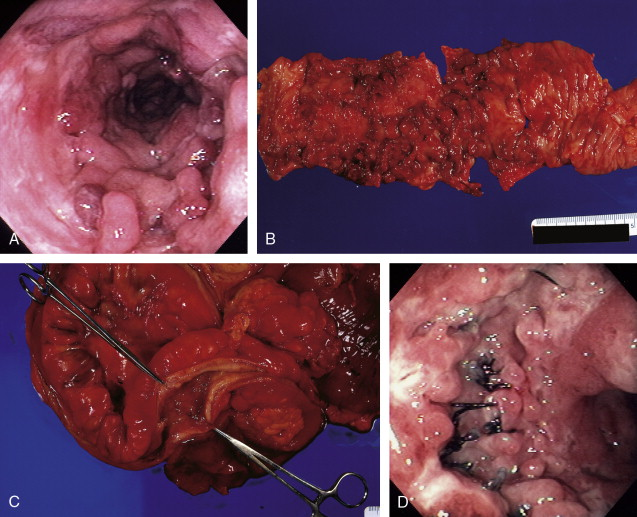Figure 5.41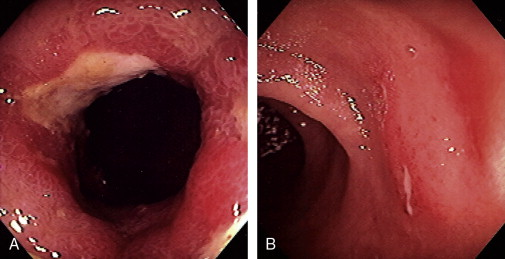Figure 5.42Figure 5.43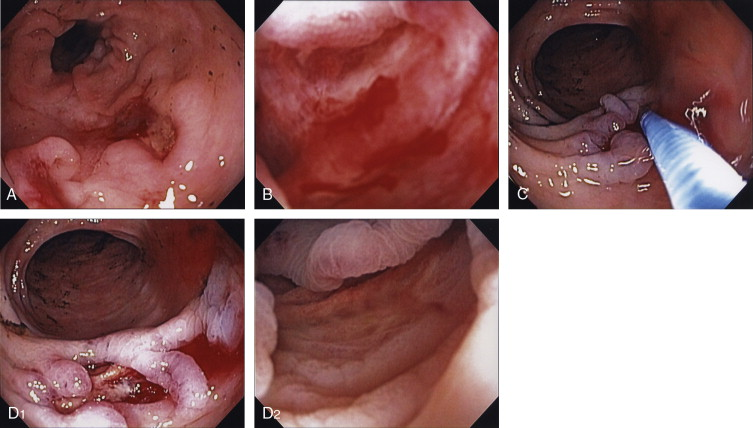Figure 5.44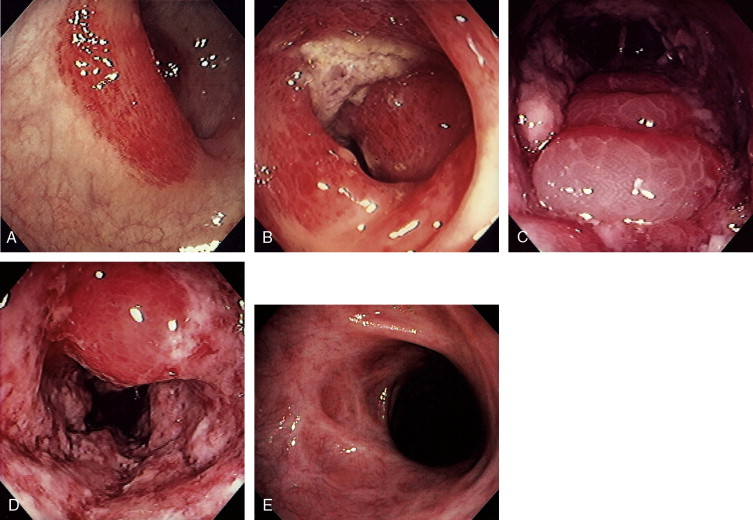Figure 5.45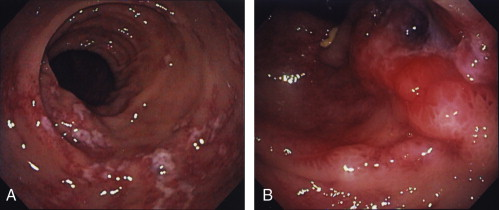Figure 5.46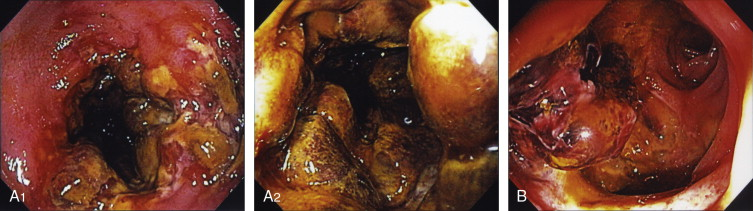Figure 5.47

Ischemic Colitis ( Figure 5.48 )

• Bacterial colitis

• Inflammatory bowel disease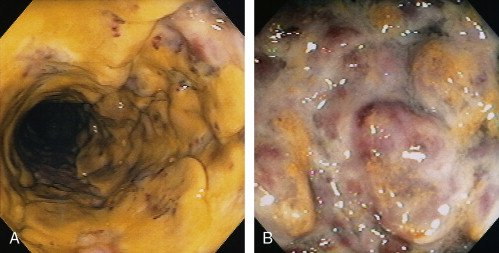Figure 5.48

Differential DiagnosisFigure 5.49Figure 5.50Figure 5.51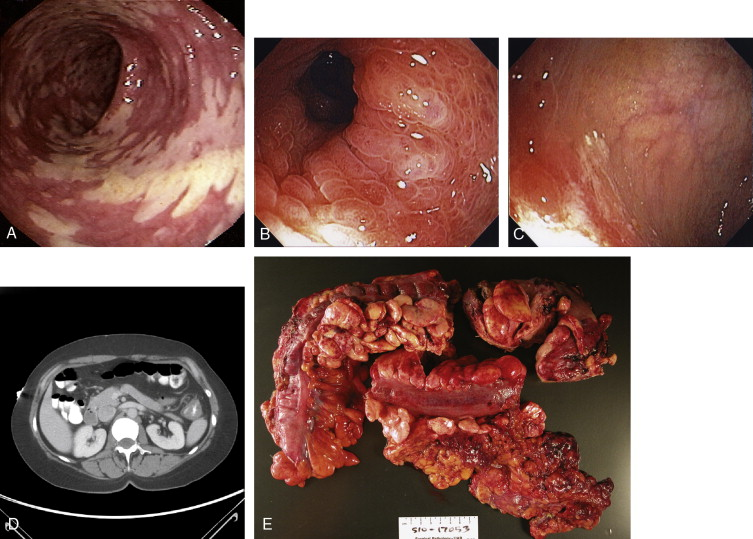Figure 5.52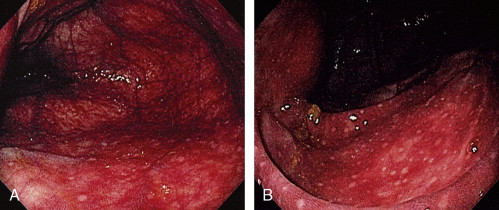Figure 5.53Figure 5.54Figure 5.55Figure 5.56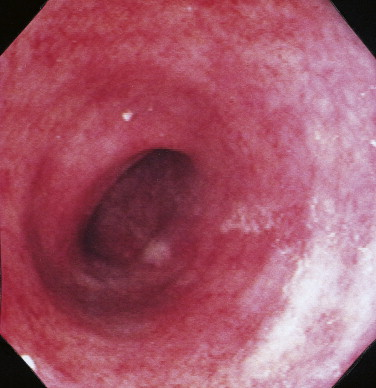Figure 5.57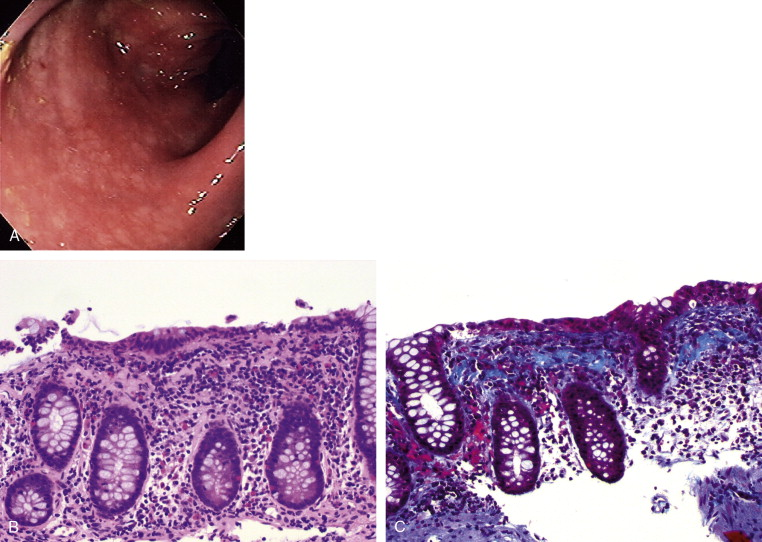Figure 5.58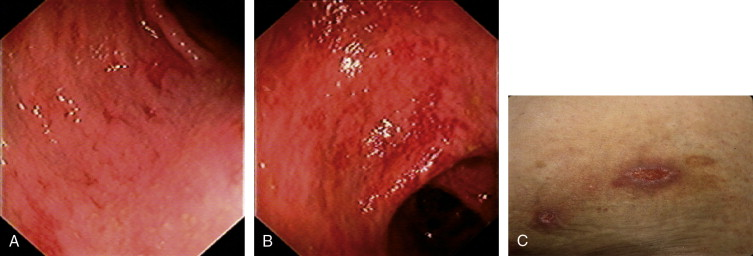Figure 5.59Figure 5.60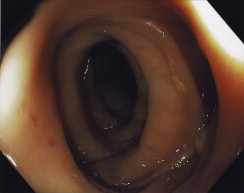Figure 5.61Figure 5.62Figure 5.63Figure 5.64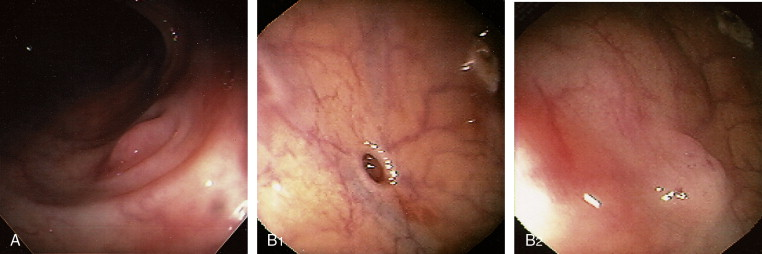Figure 5.65
Feb 5, 2019 | Posted by in GASTROENTEROLOGY | Comments Off on Colon
Premium Wordpress Themes by UFO Themes